# Meta-analysis

## 2005-03-18

Meta-analysis is the quantitative combination of results from multiple research studies. There are three steps in a typical meta-analysis model.

Extract individual estimates and standard errors from each study

Combine these estimates using a fixed or random effects model

Display the results graphically.

This page uses resources originally developed on my weblog: November 29, 2004, January 12, 2005, February 25, 2005, and March 11, 2005. I also have a web page about the special problems associated with a meta-analysis for a diagnostic test and a non-technical introduction on the practical interpretation of a meta-analysis.

Step 1. Extract individual estimates.

When you look at the individual summaries in a meta-analysis, they will report the results in a variety of ways. You need to extract these results in a common format, and the process depends a lot on the type of outcome being reported.

For a continuous outcome, a commonly reported statistic is the difference between the treatment mean and the control mean divided by the standard deviation in the control group.For this equation and all equations below, the subscript iT represents data from the treatment group of the ith study and the subscript iC represents data from the control group of the ith study.

It seems a bit unusual to use the standard deviation just from the control group. The rationale is that if you have two or more treatments in a study compared to control, the denominator never changes when you use just the control group standard deviation.

There are some variations on this formula that use a pooled variance estimate or that adjust for biases due to small sample sizes.

The standard error of the estimate is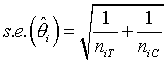For a binary outcome, such as mortality, you have several choices. You can compute the risk differenceYou can also compute the relative risk, but traditionally, this is transformed to the log scale first.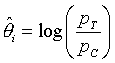You can also compute the odds ratio, and this is almost always transformed to the log scale as well.The standard error of the risk difference isFor the relative risk and the odds ratio, we need to analyze the data on the log scale. The log relative risk has a standard error of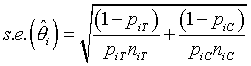and the log odds ratio has a standard error ofThere is no consensus on the best measure among the risk difference, relative risk, or odds ratio. The risk difference has certain advantages in interpretability, but the log odds ratio often has fewer problems with heterogeneity.

Step 2. Compute a preliminary estimate of overall effect.

Now that you have all the data together, the first thing you want to do is to combine it. In a perfect world, you would think carefully about your studies and the particular meta-analysis model that you want and whether it makes sense to compute any combined estimate at all. Only after a lot of careful thought would you proceed.

But let’s be realistic. You and I are both impatient, so we want to see right away what is going on. So go ahead and compute a simple estimate of combined effect. Don’t get emotionally attached to that estimate, because a better choice might be a more complex estimate or possibly no estimate at all.

The simplest combined estimate is a weighted average of the individual study results. The weights are inversely proportional to the square of the standard error,which gives greater weight to those studies with smaller standard errors. The weighted average iswhere r is the number of studies in the meta-analysis. This is known as the fixed effects estimate. It is a good starting point for further analysis, but after you have taken a careful look at this estimate and the individual studies that go into producing this estimate, you may decide to use a different estimate or dispense entirely with estimating an overall effect.

The formulas for confidence limits for this estimate are simple enough, but I won’t present them here.

Example: A meta-analysis of inhaled steroid use in chronic obstructive pulmonary disease:

• Effects of inhaled corticosteroids on sputum cell counts in stable chronic obstructive pulmonary disease: a systematic review and a meta-analysis. Gan WQ, Man SP, Sin DD. BMC Pulm Med 2005: 5(1); 3.

showed standardized mean differences (smd) for the reduction in Total Cell counts and confidence limits (lcl, ucl) in six studies in Table 3. I retyped that data in SPSS.I computed the standard error by subtracting the lower confidence limit from the standardized mean difference and then divided by 1.96. I also computed as the inverse of the squared standard error to represent the weight for each study.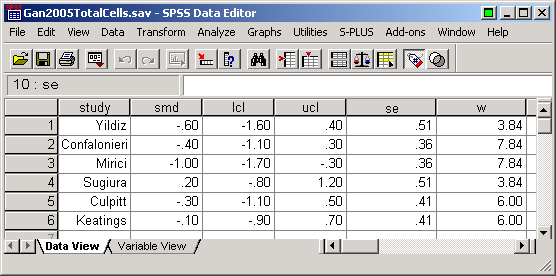The sum of the weights is 35.37 and the sum of smd times the weights is -14.91. Divide the second value by the first to get the overall estimate of -0.42. The fixed effects standard error for the overall estimate is 0.17 and a 95% confidence interval is -0.09 to -0.75.

Another example of a meta-analysis appears in

• Acetylcysteine for prevention of contrast-induced nephropathy after intravascular angiography: a systematic review and meta-analysis. Bagshaw SM, Ghali WA. BMC Med 2004: 2(1); 38.

I re-typed the table of odds ratios and 95% confidence intervals into Microsoft Excel.To calculate a standard error, you first have to transform the odds ratio and the confidence limits to the log scale. I used base 10 logarithms, here but any other type of logarithm will also work.To compute a standard error, take the log(ucl), subtract the log(or) and divide by 1.96. I could have used the log(lcl) instead, but if you look at the original data, some of the lower limits are 0.01 and 0.02. I was worried that there might be a lot of rounding error in those values, since only one significant figure is displayed.

Next, I computed weights and a weighted sum.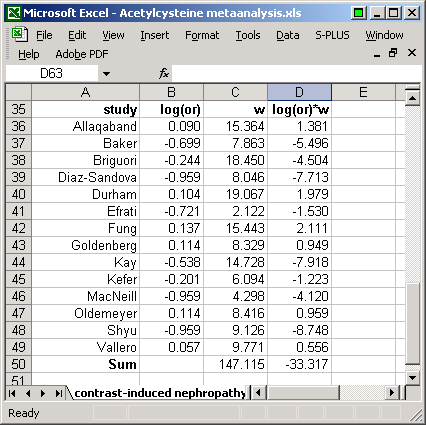The overall estimate of the log odds ratio is -33.317 / 147.115 = -0.226. Take the inverse of the sum of the weights and calculate a square root to get a standard error for this combined estimate (0.082). A 95% confidence interval on the log scale is -0.387 to -0.065. Transforming this back to the original scale of measurement gives you an overall odds ratio of 0.59 and confidence limits of 0.41 to 0.86.

Most commonly used statistical software does not include programs for meta-analysis. You can download special user contributed libraries for meta-analysis for Stata and for R.

Here is an example of an R program, plus the output using the meta library.

f0 <- TotalCells.ma <- "X:/webdata/TotalCells.csv"
attach(Cells.dat)
library(meta)
Cells.ma <- metagen(TE=Cells.smd,seTE=Cells.se,studlab=study,sm="SMD")
print(Cells.ma)
plot(Cells.ma,comb.f=T)

SMD                   95%-CI %W(fixed) %W(random)
Yildiz       -0.6 [-1.5996;  0.3996]     10.98      10.98
Confalonieri -0.4 [-1.1056;  0.3056]     22.03      22.03
Mirici       -1.0 [-1.7056; -0.2944]     22.03      22.03
Sugiura       0.2 [-0.7996;  1.1996]     10.98      10.98
Culpitt      -0.3 [-1.1036;  0.5036]     16.99      16.99
Keatings     -0.1 [-0.9036;  0.7036]     16.99      16.99

Number of trials combined: 6

SMD             95%-CI       z p.value
Fixed effects model  -0.4203 [-0.7515; -0.0891] -2.4874  0.0129
Random effects model -0.4203 [-0.7515; -0.0891] -2.4874  0.0129

Quantifying heterogeneity:
tau^2 = 0; H = 1 [1; 1.96]; I^2 = 0% [0%; 74.1%]

Test of heterogeneity:
Q d.f. p.value
4.9    5  0.4287

Method: Inverse variance method


Notice that there is no difference between the random effects model and the fixed effects model. That is because for this data set, there is no evidence of heterogeneity. The Cochran’s Q value is smaller than the degrees of freedom and the estimate of tau-squared is zero.

Here’s what the analysis of the Acetylcysteine data would look like using R and the meta library.

f0 <- "X:/webdata/Acetylcysteine1.csv"
attach(acetyl.dat)
log.or <- log(or)
se <- (log(ucl)-log.or)/1.96
acetyl.ma <- metagen(TE=log.or,seTE=se,studlab=study,sm="OR")
print(acetyl.ma)

OR           95%-CI %W(fixed) %W(random)
Allaqaband   1.23 [0.3889; 3.8899]     10.44       9.18
Baker        0.20 [0.0400; 1.0000]      5.34       6.41
Briguori     0.57 [0.1993; 1.6300]     12.54       9.93
Diaz-Sandova 0.11 [0.0224; 0.5400]      5.47       6.50
Durham       1.27 [0.4518; 3.5699]     12.96      10.06
Efrati       0.19 [0.0086; 4.2098]      1.44       2.40
Fung         1.37 [0.4345; 4.3199]     10.50       9.20
Goldenberg   1.30 [0.2721; 6.2098]      5.66       6.64
Kay          0.29 [0.0895; 0.9400]     10.01       9.00
Kefer        0.63 [0.1013; 3.9199]      4.14       5.44
MacNeill     0.11 [0.0125; 0.9700]      2.92       4.24
Oldemeyer    1.30 [0.2744; 6.1598]      5.72       6.68
Shyu         0.11 [0.0247; 0.4900]      6.20       7.01
Vallero      1.14 [0.2691; 4.8299]      6.64       7.29

Number of trials combined: 14

OR           95%-CI       z p.value
Fixed effects model  0.5937 [0.4092; 0.8612] -2.7468   0.006
Random effects model 0.5428 [0.3231; 0.9121] -2.3076   0.021

Quantifying heterogeneity:
tau^2 = 0.4187; H = 1.35 [1; 1.84]; I^2 = 44.9% [0%; 70.5%]

Test of heterogeneity:
Q d.f. p.value
23.6  13    0.035

Method: Inverse variance method


One important thing to note is that R expects you to use natural logarithms (base e) rather than base 10 logarithms. When I first did this, I used base 10 logarithms and all the results were too small.

A common way to display the individual study results and a combined estimate of effects is a graph known as a forest plot. An example of a forest plot appears in

and because this is an open-access article, I can reproduce the graph here.Since BMC Medicine is published with an open access license, I can freely reproduce this image, as long as I cite the source.

I was always confused by the funny squares in a forest plot, so I looked for a description. Here is what the User’s Guide for RevMan (software created by the Cochrane Collaboration) says about forest plots:

The graph is a forest plot where the confidence interval (CI) for each study is represented by a horizontal line and the point estimate is represented by a square. The size of the square corresponds to the weight of the study in the meta-analysis. The confidence interval for totals are represented by a diamond shape. The scale used on the graph depends on the statistical method. Dichotomous data (except for risk differences) are displayed on a logarithmic scale. Continuous data and risk differences are displayed on a linear scale. Generic inverse variance data are displayed on either a logarithmic scale or a linear scale depending on the settings in RevMan.http://www.cc-ims.net/download/revman/Documentation/User%20guide.pdf (page 36).

Here is an example of the Forest plot, as drawn by R and the meta library.

> plot(TotalCells.ma,comb.f=T)`Another way to display the results of a meta-analysis looks at the cumulative effect over time as additional studies accumulate. At the top of the graph, you display the confidence interval for the estimate from the first study published. Directly below that you display the confidence interval for the combined effect of the first and second studies. Below that is the combined effect of the first, second, and third studies, and so forth. An example of this cumulative display appears in

shows cumulative meta-analysis, which is the cumulated effects over time of studies in the use of erythropoietin (EPO) to treat cancer related anemia.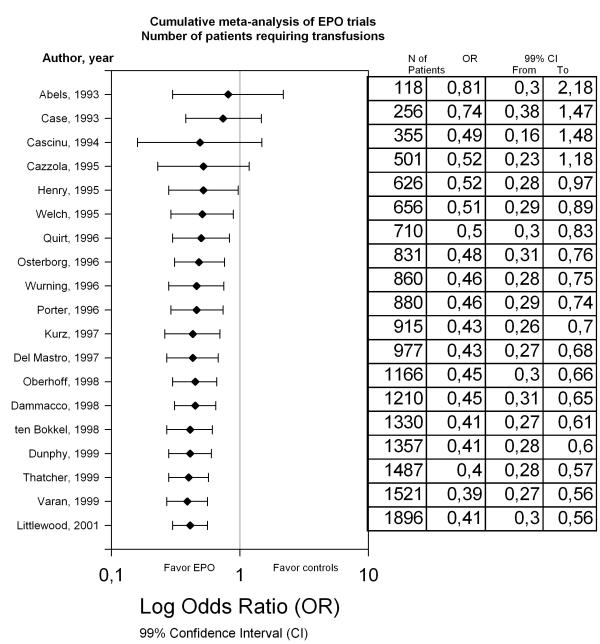Since BMC Cancer is published with an open access license, I can freely reproduce this image, as long as I cite the source.

The outcome variable, the odds ratio for whether a patient requires transfusion, showed a significant benefit for EPO. It also shows that sufficient evidence had already accumulated by 1995 to demonstrate this benefit. If such a meta-analysis had been performed back then, there would have been no need to run the additional trials. These redundant trials are bad because they wasted scarce research dollars on a topic where sufficient information had already been accumulated to answer the research question. They are also bad because half of the patients in these post-1995 trials received no treatment or placebo, even though there was enough evidence at that time to show that this is an inferior option.

Some have suggested that any protocol submitted to an Institutional Review Board (IRB) should include a systematic overview or meta-analysis of the previous research (see Chalmers 1996), rather than just a simple literature review, to prevent future IRBs from making the same mistake of those that approved the post-1995 studies of EPO. In some situations, that is definitely overkill, but it is a suggestion worth serious consideration in other circumstances.

Step 3. Evaluate the studies for publication bias and heterogeneity.

After you have an overall estimate, you should compute the amount of variability of each study from the overall estimate. You do this by computing a Z-score for each study,and then seeing how much all of these Z-scores differ from zero by squaring the Z-scores and adding them up. This gives you a test statistic, Cochran’s Q,An unusually large value for Q implies substantial heterogeneity, because you have more variation among the studies than you would expect just by looking at the individual standard errors. If there is no heterogeneity, then Q should be approximately equal to r-1, which implies that the squared Z-scores are, on average, just slightly less than 1.

Many experts have rejected the use of quantitative measures such as Cochran’s Q for assessing heterogeneity and suggest instead that you examine the studies qualitatively and provide a subjective assessment of the degree of heterogeneity among the research studies.

Another alternative is I-squared (Higgins 2003), a statistic that measures the proportion of inconsistency in individual studies that cannot be explained by chance.Negative values are not allowed for I-squared. If you compute a negative value, set I-squared to zero instead.

I-squared is bounded above by 100% and values close to 100% represent very high degrees of heterogeneity.

This measure is preferred to Cochran’s Q. The problem with Cochran’s Q, the authors claim, is that it tends to have too little power with a collection of studies with small sample sizes and too much power with a collection of studies with large sample sizes. Values of I-squared equal to 25%, 50%, and 75% representing low, moderate, and high heterogeneity, respectively.

The random effects model is an alternative way to combine estimates that explicitly accounts for heterogeneity. In the random effects model, each study statistic is assumed to be composed of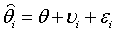where the second component is normally distributed random effectthat accounts for the heterogeneity from study to study. A frequent criticism of the random effects meta-analysis is this assumption that the random effects follow a bell shaped curve. There is some suggestion that perhaps heterogeneity manifests itself as a bimodal distribution instead.

You can use the Method of Moments and Cochran’s Q statistic to estimate the between study variation:Notice that the numerator is a measure of how much the Cochran’s Q statistic exceeds its degrees of freedom. If you get a negative estimate here, simply replace it with an estimate of zero.

With an estimate of between study variation, you can now compute the random effects estimate as a weighted average, just like the fixed effects estimate, except the weights in the random effects estimate are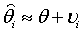where w~i~ are the weights used in the fixed effects model.

These weights are going to be closer to uniform or equal weighting than the weights in a fixed effects model. If you think about it long enough, this is actually quite intuitive. In a model where the study heterogeniety is large, large enough to dominate the standard errors, you effectively have a random sample of studies each of which is more or less identically distributed:In addition to producing weights that are closer to equal weighting, the confidence intervals for a random effects meta-analysis are typically wider than a fixed effects meta-analysis because the estimated study heterogeneity adds an additional source of uncertainty to the confidence interval calculations.

The funnel plot is a graphical exploration of the study results looking for evidence of publication bias. An example of a funnel plot appears in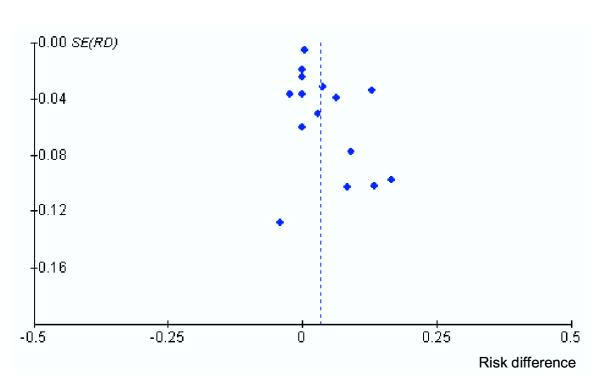Another funnel plot with conical guidelines superimposed appears in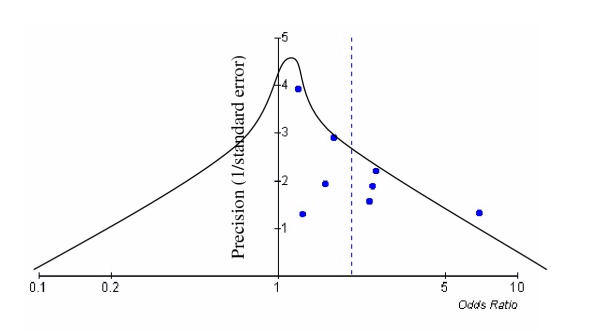Interestingly enough, most of the meta-analyses published in Biomed Central had the following statement (almost word for word)

Publication bias was not assessed using funnel plots as these tests have been shown to be unhelpful.

These articles then cited the following two references

I have not yet read these articles, but I would agree that the funnel plot is often difficult to interpret. There are some numerical summary measures that try to quantify the departure from symmetry in the funnel plot, but these measures may also have problems.

The trim and fill method uses the funnel plot to try to estimate the missing unpublished studies. In this approach, studies that are asymmetrically distributed (that have no matching study on the opposite side of the funnel plot) are removed from the plot. Then the funnel plot is filled in using symmetric pairs from the trimmed study. This produces a funnel plot with extra imputed studies that make the plot symmetric. The trim and fill method is quite controversial and should be considered an exploratory approach. If, for example, you use this method and the overall estimate changes by a trivial amount, then you have indirect evidence that publication bias did not seriously influence your outcome.